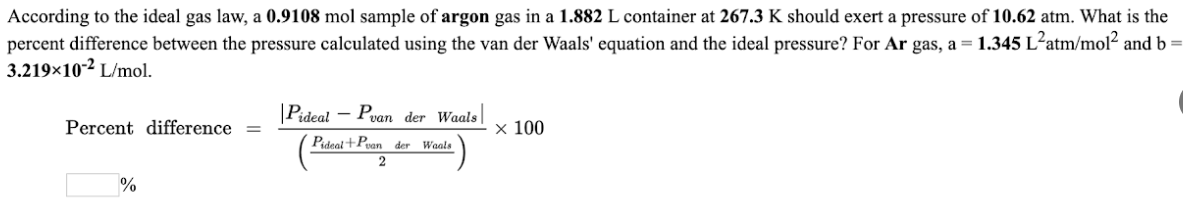# According to the ideal gas law, a 0.9108 mol sample of argon gas in a 1.882 L container at 267.3 K should exert a pressure of 10.62 atm. What is the percent difference between the pressure calculated using the van der Waal's equation and the ideal pressure? For Ar gas, a = 1.345L^2atm/mol^2 and b = 3.219x10^-2 L/mol.